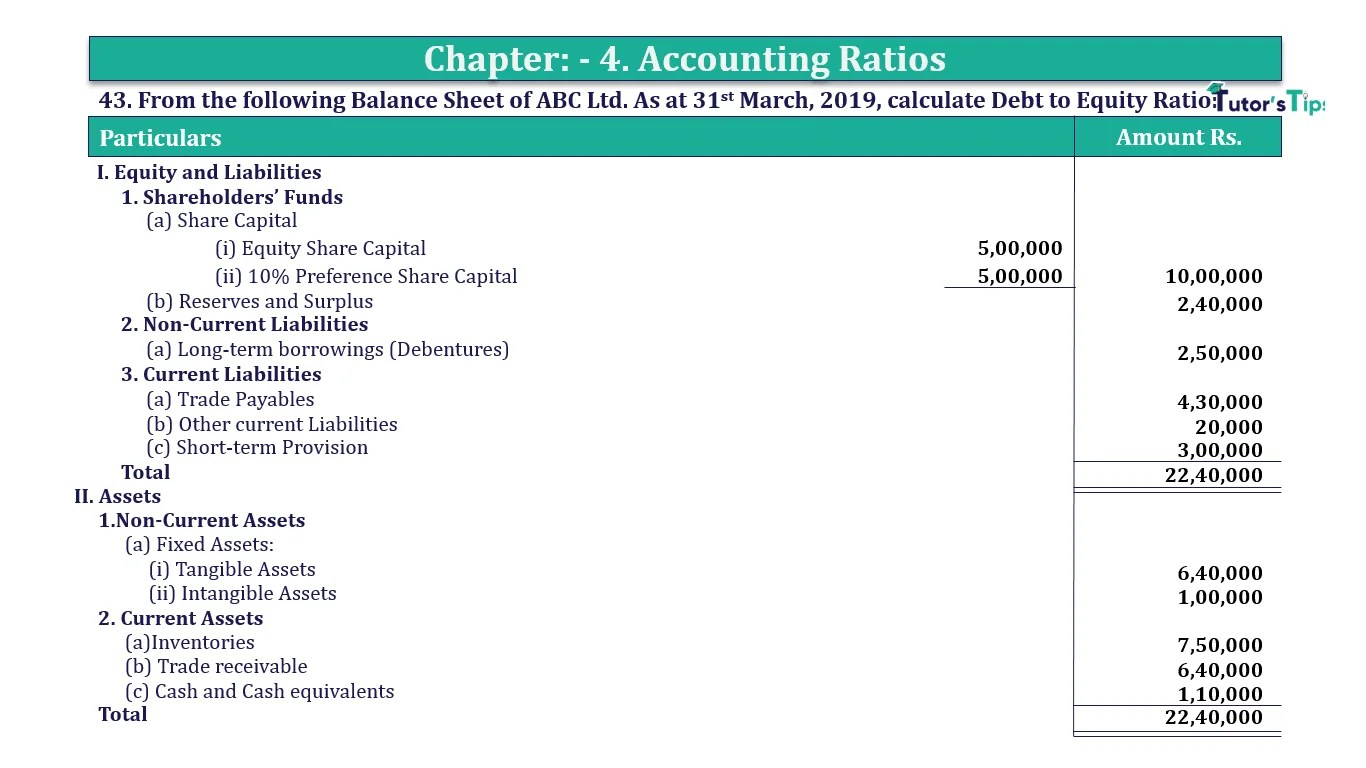# Question 43 Chapter 4 of +2-B – T.S. Grewal 12 ClassQuestion No. 43- Chapter No.4 - T.S. Grewal +2 Book Part B

Question 43 Chapter 4 of +2-B

43. From the following Balance Sheet of ABC Ltd. As at 31st March 2019, calculate Debt to Equity Ratio:

 Particulars Amount Rs. I. Equity and Liabilities 1. Shareholders’ Funds (a) Share Capital (i) Equity Share Capital 5,00,000 (ii) 10% Preference Share Capital 5,00,000 10,00,000 (b) Reserves and Surplus 2,40,000 2. Non-Current Liabilities (a) Long-term borrowings (Debentures) 2,50,000 3. Current Liabilities (a) Trade Payables 4,30,000 (b) Other current Liabilities 20,000 (c) Short-term Provision 3,00,000 Total 22,40,000 II. Assets 1.Non-Current Assets (a) Fixed Assets: (i) Tangible Assets 6,40,000 (ii) Intangible Assets 1,00,000 2. Current Assets (a)Inventories 7,50,000 (b) Trade receivable 6,40,000 (c) Cash and Cash equivalents 1,10,000 Total 22,40,000

### The solution of Question 43 Chapter 4 of +2-B: –

 Equity = Equity Share Capital + 10% Preference Share Capital + Reserves and Surplus = Rs. 5,00,000 + Rs. 5,00,000 + Rs. 2,40,000 Equity = Rs. 12,40,000 Debt = Long-Term Borrowings(Debentures) Debt = Rs. 2,50,000

 Debt to Equity Ratio = Debts = Rs. 2,50,000 Equity Rs.12,40,000 = 0.2: 1

Balance Sheet: Meaning, Format & Examples

Comment if you have any question.

Also, Check out the solved question of previous Chapters: –